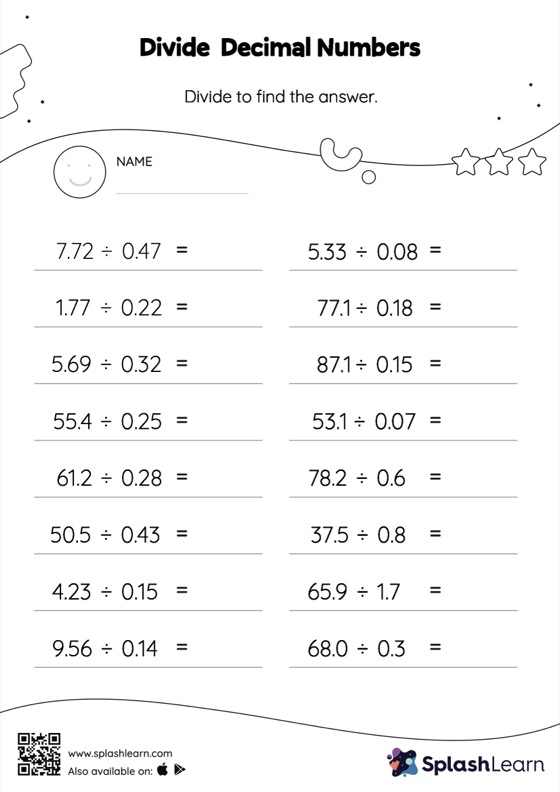# Divide Decimal Numbers with Remainder: Horizontal Division Worksheet

Home > Divide Decimal Numbers with Remainder: Horizontal DivisionWhen students divide two decimals, they first divide as if there is no decimal. Depending on the number of decimal digits in each number, they add a decimal point at the appropriate location in the quotient. Students practice this concept in great detail with this divide decimal numbers with remainder worksheet. As the worksheet uses a horizontal format, it allows for more creativity when it comes to how the student solves the problem. In contrast, in the vertical format, students usually employ the standard method to solve.